## Sunday, 11 September 2011

### Determinants for M2 III: more cases on factorization

1) Transpose of a matrix/determinants from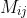to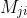is defined by: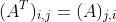﻿
The element in the i row j column is moved to j row i column.
For simplicity, you can imagine that ﻿the determinant is flipped along the principal diagonal line (which is the top-left to bottom-right one).
Example: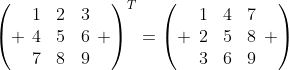Even if it isn't square matrix it still works: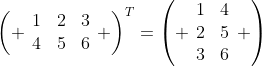In HKDSE, transpose is almost useless so I won't discuss its main properties, except one: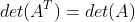It may help when we can't solve a determinants, flipping it gives more clues. Take the HW as example:
Example: Factorize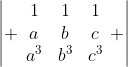, hence evaluate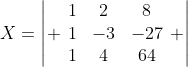.
Now notice that when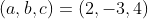, the original one is the transpose of X.
Therefore,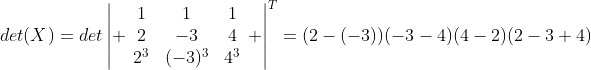(the factorization has been demonstrated before) which gives the answer -210.

Note that transpose has given the symmetricities between rows and columns.

Uniqueness of degree in determinants

Sometimes the factorization does not give a unique degree in the polynomial expression which may cause difficulties in factorization.
Recall that determinants is the sum of product of elements from distinct rows and columns. Therefore it each row or column has unique degree, the factorization result has a unique degree.
For example,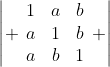In such case you can treat 1 as a variable in order to equate the degree. The determinant becomes:Note that this determinant is NOT cyclic because each row are not in cyclic relationship.
Cyclic group of (a,b,c): (a,b,c) -> (b,c,a) -> (c,a,b) -> (a,b,c)
Cyclic group of (b,a,c): (b,a,c) -> (a,c,b) -> (c,b,a) -> (b,a,c)
They are two different group, and in this example, (c,a,b) can't reach (a,c,b) by cyclic relationship.
However we see that (a,b) is symmetrical becauseTherefore if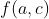is a factor of the det.,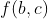is also a factor of the det.
Notice that when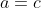,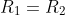,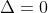(the value of determinant = 0), so (c-a) is a factor of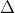. As we have proven, (c-b) is also a factor of.
Though it's not cyclic, each row still contain a,b,c, which tell us (a+b+c) is a factor of it (this is an usual and important practice in factorizing determinants).
Equating degree,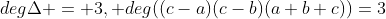, so we have factorized out all factor of, we now assume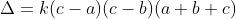, where k is a constant. By equating the coefficient, we get k = 1, and putting c = 1, we have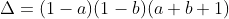Case for x=y=z

When two equal variables "kill" the determinant, we say (a-b) is a factor. How to determine the factor when the condition "x=y=z" kill the determinant?

If x=y=z is the condition to make determinant zero, then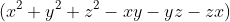is the factor of the determinant.

Prove: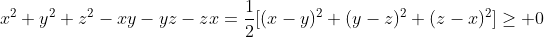. It's zero only if x=y=z, and therefore by factor theorem it's a factor of the determinants.

This is a simplified version of AM-GM inequalities or Rearrangement Inequalities, HKALE players should be familiar with it.

Example:
Factorize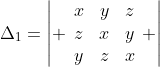, hence show that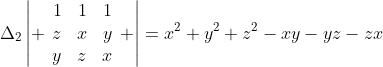Step 0: Notice that the determinant is cyclic.
Step 1: Notice that the determinant vanishes when x=y=z, sois a factor of the determinant.
Step 2: Equating degree, the degree of determinant is 3 while the current factor is 2, that remains one more factor with degree 1, then that must be (a+b+c).
Step 3: Equating coefficient,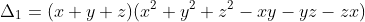Step 4: The second part of the question: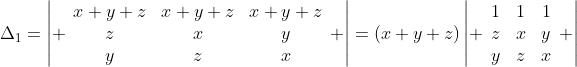Therefore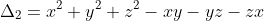.

For ugly result which can't be factorized

In this case we can't do much other than directly expansion.
Example: express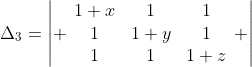in polynomial.
Notice that
1) This is symmetrical (as we have proven)
2) Each term of the polynomal does not contain a term with a square or a cube of a certain variable like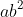.
Then we can conclude that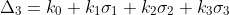, where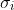are elemental functions for 3 variables.
By equating the coefficient, we have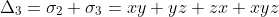.

Example: express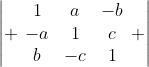in polynomial.
Notice that: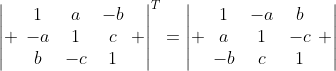, therefore the sign of variable does change the value of determinant. Since the degree is under 3, we deduce that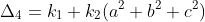where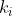are constants. By equating the coefficient, we have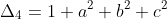.

Exercise:
1)Show that for distinct a,b,c,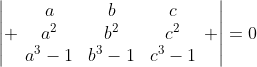, then abc=1.
2)Factorize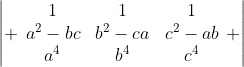given that the product is four linear and one quadratic factors. [Sheffield past paper]
3)Factorize.
4)Show that (a,c) is symmetrical for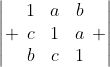, hence express it in polynomial.
5)Show that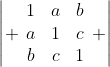is symmetrical, hence express it in polynomial.
6)Why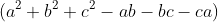is not a factor of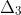?

Extra question:
For a square matrixwhereandare constants. The rest of the entries are 1. Show by induction, or otherwise,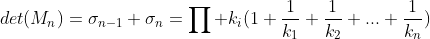.  (AL level; 6~7M)